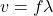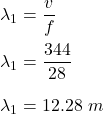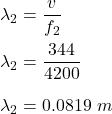## A piano emits frequencies that range from a low of about 28 Hz to a high of about 4200 Hz. Find the maximum wavelength in air attained by th

Question

A piano emits frequencies that range from a low of about 28 Hz to a high of about 4200 Hz. Find the maximum wavelength in air attained by this instrument when the speed of sound in air is 344 m/s. Answer in units of m.

in progress 0
6 months 2021-07-29T02:39:06+00:00 1 Answers 5 views 0

## Answers ( )

The maximum wavelength in air attained by this instrument is 12.28 meters.

Explanation:

Given that,

A piano emits frequencies that range from a low of about 28 Hz to a high of about 4200 Hz.

We need to find the maximum wavelength in air attained by this instrument when the speed of sound in air is 344 m/s.

We know that the speed of any wave is given by :When f = 28 HzWhen f = 4200 HzSo, the maximum wavelength in air attained by this instrument is 12.28 meters.